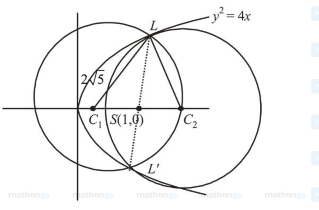# Let the latus ractum of the parabolaQuestion:

Let the latus ractum of the parabola $y=4 x$ be the common chord to the circles $C_{1}$ and $C_{2}$ each of them having radius $2 \sqrt{5}$. Then, the distance between the centres of the circles $C_{1}$ and $C_{2}$ is :

1. (1) $8 \sqrt{5}$

2. (2) 8

3. (3) 12

4. (4) $4 \sqrt{5}$

Correct Option: , 2

Solution:Distance between the centres

$=C_{1} C_{2}=2 C_{1} S=2 \sqrt{20-4}=8$